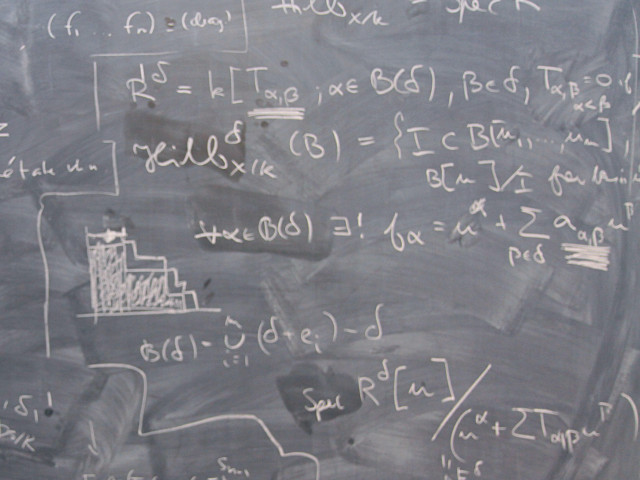# SF1630 Discrete Mathematics 9.0 credits

#### Diskret matematikBasic course of discrete mathematics and algebra.

### Offering and execution

Course offering missing for current semester as well as for previous and coming semesters

## Course information

### Content and learning outcomes

#### Course contents *

Linear recursion with constant coefficients. The Master theorem.

Graphs. Euler circuits, Hamilton cycles. Trees. Graph coloring. Planar graphs. Euler's polyhedron formula, Kuratowski's theorem. Bipartite graphs. Hall's marriage theorem. Augmenting alternating paths. Transversals.

Integer arithmetic. Divisibility. Euclid's algorithm for the greatest common divisor. Linear Diophantine equations with two unknowns. Unique factorization. Modular arithmetic. Chinese remainder theorem. Euler's ɸ- and Möbius' μ-function. Euler's theorem and Fermat's little theorem.

Bijections, injections, surjections. Cardinality. Finite, countable and uncountable sets.

Combinatorics. Pigeon principle. Addition and multiplikation principles. Different kinds of selections. Binomial numbers, multinomial numbers. Inclusion/exclusion. Partitions and equivalence relations. Stirling numbers of the second kind.

Permutations. Cycle notation. Conjugated permutations. Even and odd permutations.

Basic group theory. Order of group elements and group. Cyclic groups. The symmetric group. Subgroups, cosets. Lagrange's theorem. Group actions on sets. Burnside's lemma.

Rings and fields. Factorization of polynomials. Irreducible polynomials. Finite fields.

Error correcting linear binary codes. RSA cryptosystem. Primality tests.

#### Intended learning outcomes *

The general objective is to give basic knowledge in Discrete Mathematics, especially in the solution of combinatorial problems, the knowledge of some important algebraic structures and basic knowledge of graph theory. Also the ability to perform a stringent mathematical discussion is trained.

After passing the course a student should be able to

• formulate basic theorems and definitions of important concepts in discrete mathematics,
• apply theorems and methods in discrete mathematics.

#### Course Disposition

No information inserted

### Literature and preparations

#### Specific prerequisites *

SF1604 Linear algebra II (or equivalent)

#### Recommended prerequisites

No information inserted

#### Equipment

No information inserted

#### Literature

Biggs: Discrete Mathematics, 2:nd ed.

Hand-out material.

### Examination and completion

A, B, C, D, E, FX, F

#### Examination *

• TENA - Examination, 6.0 credits, Grading scale: A, B, C, D, E, FX, F
• TENB - Examination, 3.0 credits, Grading scale: A, B, C, D, E, FX, F

Based on recommendation from KTH’s coordinator for disabilities, the examiner will decide how to adapt an examination for students with documented disability.

The examiner may apply another examination format when re-examining individual students.

#### Other requirements for final grade *

Two written exams (TENA; 6 credits), (TENB; 3 cr).

#### Opportunity to complete the requirements via supplementary examination

No information inserted

#### Opportunity to raise an approved grade via renewed examination

No information inserted

### Further information

#### Course web

Further information about the course can be found on the Course web at the link below. Information on the Course web will later be moved to this site.

Course web SF1630

SCI/Mathematics

#### Main field of study *

Mathematics, Technology

First cycle